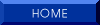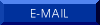Pafnuti Chebyshev

b. 16 (4) May 1821
d. 8 December (26 November) 1894, St. Petersburg

Chebyshev began mathematical studies at Moscow University in 1837 under Brashman. An address given in 1841 by Brashman seems to have been the catalyst for Buniakovsky's book Foundations of the Mathematical Theory of Probabilities (1846) and Chebyshev's own master's thesis ``An Essay on the Elementary Analysis of the Theory of Probability" (1845). Unable to find a teaching position in Moscow,  he took up work as a lecturer at St. Petersburg University the next year. After defending his work "The Theory of Congruences" he was awarded a doctorate in 1849.

At St. Petersburg, Chebyshev became acquainted with Buniakovsky. Between 1850 and and 1859, Bunkiakovsky lectured on probability, but thereafter, until retirement in 1882, Chebyshev delivered the course.  He is known as the founder of the St. Petersburg school of probability and may count among his students Lyapounov and Markov. In fact, we possess lecture notes taken down by Lyapounov (translated into English by Sheynin) during the year 1879-1880, by Artemiev in 1876-1878 and some very condensed notes by Markov written at some other time. The notes of Lyapounov were analyzed by Oscar Sheynin in "Chebyshev's lectures on the theory of probability," Archive for History of the Exact Sciences (1994) Volume 46, No. 4, p. 321-340.

Chebyshev is known for creation of the Chebyshev polynomials (1854), the Bienaymé-Chebyshev Inequality (1867) of probability theory and a proof of the Central Limit Theorem (1887).

His collected works were published in two volumes as Oeuvres de P.L. Tchebychef at St.-Petersburg in 1899 and 1907 respectively. Those papers previously appearing only in Russian were translated into French for this edition. Our renderings into English are derived from these volumes.

A brief biography is given in Statisticians of the Centuries editied by Heyde and Seneta, 2001.

Probability papers

 "Demonstration élémentaire d'une proposition générale de la théorie des probabilités," Crelle, Journal für die reine und angewante Mathematik, B. 33, p.259-267.This paper concerns Bernoulli's Theorem or Weak Law of Large Numbers.

 "Des valeurs moyennes," Liouv. J. Mth. 2nd series, 12, pp. 177-184. Here may be found the Bienaymé-Chebyshev Inequality. But see also Bienaymé's paper of 1853: "Considérations à l'appui de la découverte de Laplace sur la loi de probabilité dans la méthode des moindres carrés," in which he too developed the inequality. Original in Mat. Sbornik, 2 (2), 1-9. See also A Source Book in Mathematics by D.E. Smith, pp. 580-7.

 "Sur les valeurs limites des intégrales," Liouville, Journal de mathématiques pures et appliquées. II series, T. XIX, p. 157-160. Read to the Congress of the French Association for the Advancement of the Sciences, at Lyon. Session of 27 August 1873.

 "Sur deux théorèmes relatifs aux probabilités," Acta mathematica, T. XIV (1890-1891), p. 805-815. Read 10 March 1887. Here may be found his proof of the Central Limit Theorem.

Interpolation by Method of Least Squares

 "Théorie des méchanisms connus sous le nom de parallélogrammes," Mémoires Présentés a l'Académie Impériale des Sciences de St. Pétersbourg par Divers Savants 7, pp. 539-568. In Oeuvres de P.L. Tchebychef, Vol. 1, pp. 111-143.

 "Sur une formule d'analyse," Bulletin physico-mathématique de l'Académie Impériale des sciences de St.-Pétersbourg. T. XIII, No. 13, p. 210-211.  Read 20 October 1854.

 "Extrait d'un Mémoire sur les fractions continues," Bulletin physico-mathématique de l'Académie Impériale des sciences de St.-Pétersbourg. T. XIII, p. 287-288.  Read 12 January 1855.

 "Les fractions continues," Journal de mathématiques pures et appliquées. II series, T. III, p. 289-323. This was translated from the Russian by Bienaymé.

 "Sur l'interpolation des valeurs fournies par les observations," Bulletin physico-mathématique de l'Académie Impériale des sciences de St.-Pétersbourg. T. XVI, p. 353-358. Read 18 (30) March 1858. See the paper below published in the Memoirs.

 "Sur une nouvelle série," Bulletin physico-mathématique de l'Académie Impériale des sciences de St.-Pétersbourg. T. XVII, p. 257-261.

 "Sur l'interpolation dans le cas d'un grand nombre de données fournies par les observations," Mémoires de l'Académie Impériale des sciences de St.-Pétersbourg. VII series. T. I, No. 5, p. 1-81. The notice in the bulletin presented above, summarizes the paper. Here we present only the first section:
"When the number of given values surpass the one of the terms that one conserves in their expression, interpolation is able to be executed by diverse methods. But these methods, in each particular case, are far from being equally advantageous; they differ among themselves either by the prolixity more or less great of the calculations, or by the magnitude of the mean error to fear,  as long as there is concern of interpolation of the values furnished by the observations  and consequently affected of errors. As one is not able to gain beyond a certain limit under one of these relations without loss under the other, it is impossible to give a method of interpolation which is in general preferable to all the others; for, according  to the cases, one holds more either to the simplification of the calculations, or to the precision of the results. If one knows only a small number of values of an interpolated function, there is presented few resources in order to attenuate the influence of their errors on the result sought, and then it is important  to draw from the data of interpolation all the part possible in order to diminish the mean error to fear, that which one is able to do only by aid of the method of least squares. In the contrary case, the considerable number of data that one has to its disposition, dispenses us of recourse to the method of least squares which requires some calculations too long. Then, for the simplification of the numerical operations, one is able also to sacrifice a part more or less considerable of that which the given values offer in order to estimate the sought result. In the Memoir Sur les fractions continues, presented to the Academy in 1855, we have treated of the parabolic interpolation according to the method of least squares, and we are arrived to a series which furnishes directly the results of one such interpolation, indispensible, as we just saw, if the number of known values of the interpolated function is rather small. In the present Memoir we will show how, according to our methods, one arrives to other formulas of interpolation which are able to replace with advantage that of which we just spoke, as much as its application, seeing the great number of given values, ceases, on the one hand, to be important, and on the other, becomes not very practical."

"Of the diverse particular cases which are able to present the interpolation according to the number more or less great of the given values, we will limit ourselves to consider the one which is the limit of all the others where the number of given values is infinite. Although, in reality, this number is never infinite, the formulas that one finds under this assumption are able to be however of a useful application; for they present the limit towards which the results of interpolation converge very rapidly, in measure as this number increases, and it will not be difficult to see, in each particular case, by what degree of approximation these formulas are susceptible according to the given values."

 "Sur l'interpolation par la méthode des moindres carrés," Mémoires de l'Académie Impériale des sciences de St.-Pétersbourg. VII series. T. I, No. 15, p. 1-24. This is a very important paper in which he compares his method of interpolation to that of Cauchy.

 "Sur le développement des fonctions à une seule variable," Bulletin physico-mathématique de l'Académie Impériale des sciences de St.-Pétersbourg. T. I, p. 193-200.

 "Sur l'interpolation." Published in the Russian Supplement to the 4th volume of the Memoirs of the Academy of Sciences, No. 5, (1864).

"In the memoir under the title "Sur les fractions continues" I have given a formula of interpolation by the method of least squares, whatever be the given values of the function to interpolate. Now I am going to demonstrate the simplifications of which that formula is susceptible in the particularly remarkable case where the known values of the function are taken for some equidistant values of the variable. In this case, the general formula of interpolation, corresponding to the formula of Lagrange, is reduced to a series, corresponding to the formula of interpolation of Newton, containing as that here in its terms the  finite differences of successive orders 1, 2, 3,... etc., that which offers, as one knows, a great advantage in applications. This series gives the expression of the quantities to interpolate under the form of polynomials of different degrees according to the number of terms one retains, the coefficients of thes e polynomials being the same that one obtains by the method of least squares in resolving all a system of equations of which the form changes when one passes from one particular assumption on the degree of the sought expression to another. It is easy to see how much  the research of such expressions is simplified by the use of our series whence they result directly and successively from all the degrees by departing from degree 0. But this circumstance is not the only advantage that one is able to draw by employing this series for interpolation; it is yet very suitable in order to show with how many terms, or, that which reverts to the same, to what degree in the sought expression we ourselves are able to restrict. In the ordinary process of interpolation by the method of least squares it is necessary for us to redo each time the calculation of all the given values by aid of a particular formula for each assumption on the degree of this formula. This is so much more painful as these calculations must be repeated many times until  one arrives to an expression representing the data with a sufficient degree of approximation, for it is difficult to guess in advance the degree of one such expression."

"Under this relation, our formula offers this important advantage that by adding successively one term after another in the expression to interpolate, we are able at the same time to see how the sum of the squares of errors diminishes successively that one commits in determining by aid of this expression all the given values, whence it is easy to deduce also the quadratic mean of these errors, according to which one is able to judge from the sufficiency of the number of terms retained in the sought expression."

 "Sur les fractions continues algébriques," Journal de mathématiques pures et appliquées. 2nd series, X, p. 353-358. This is a letter addressed to Mr. Braxchmann and read 18/30 September 1865 in the session of the Mathematical Society of Moscow.

  "Formule d'interpolation par la méthode des moindres carrés," Mém. Couronnés Acad. Belgique 21,  pp. 125-133.

 "Sur l'interpolation des valeurs équidistantes,"

 "Sur une série qui fournit les valeurs extrêmes des intégrales, lorsque la fonction sous le signe est décomposée en deux facteurs," Read 10 May 1883.

 "Sur deux théorèmes relatifs aux probabilités."  St. Petersburg, Ac. Sci. Mem. 55,  Published in the Russian 6th Supplement  to the Memoirs. Translated by I. Lyon, Acta Mathematica 14 (1890-91), pp. 305-315.